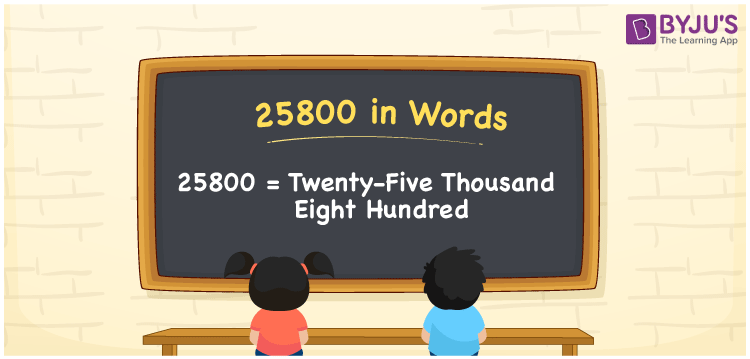# 25800 in Words

We can express 25800 in words as Twenty-Five Thousand Eight Hundred. For example, Rohit earns Rs. 25800 per month. We can write the same sentence in words as “Rohit earns Rupees Twenty-Five Thousand Eight Hundred per month”. In this article, let us learn to write a cardinal number 25800 in word form with the help of a place value chart. Also, we learn interesting facts about the number 25800 in a precise manner.

 25800 in Words Twenty-Five Thousand Eight Hundred Twenty-Five Thousand Eight Hundred in numerical form 25800

## 25800 in English Words

Generally, numbers in words are formed using the English alphabet. Thus, we can read 25800 in English as Twenty Five Thousand Eight Hundred.## How to Write 25800 in Words?

To write 25800 in words, let us create a place value chart of 5 columns since 25800 is a five-digit number. This can be shown as:

 Ten Thousand Thousands Hundreds Tens Ones 2 5 8 0 0

Thus, we can write the expanded form as:

2 x Ten Thousand + 5 x Thousand + 8 x Hundred + 0 x Ten + 0 x One

= 2 x 10000 + 5 x 1000 + 8 x 100 + 0 x 10 + 0 x 1

= 20000 + 5000 + 800 + 0 + 0

= 20000 + 5000 + 800

= 25800

= Twenty Five Thousand Eight Hundred

Hence, 25800 in words is written as Twenty Five Thousand Eight Hundred.

Interesting way of writing 25800 in words

2 = Two

25 = Twenty-Five

258 = Two Hundred and Fifty-Eight

2580 = Two Thousand Five Hundred Eighty

25800 = Twenty-Five Thousand Eight Hundred

Therefore, the word form of the number 25800 is Twenty-Five Thousand Eight Hundred.

25800 is a natural number that is the successor of 25799 and the predecessor of 25801

• 25800 in words – Twenty-Five Thousand Eight Hundred
• Is 25800 an odd number? – No
• Is 25800 an even number? – Yes
• Is 25800 a perfect square number? – No
• Is 25800 a perfect cube number? – No
• Is 25800 a prime number? – No
• Is 25800 a composite number? – Yes

## Frequently Asked Questions on 25800 in Words

### Write 25800 in words.

25800 in words is written as Twenty-Five Thousand Eight Hundred.

### Simplify 15000 + 10800, and express in words.

Simplifying 15000 + 10800, we get 25800. Hence, 25800 in words is Twenty-Five Thousand Eight Hundred.

### Is 25800 a perfect square number?

No, 25800 is not a perfect square number.